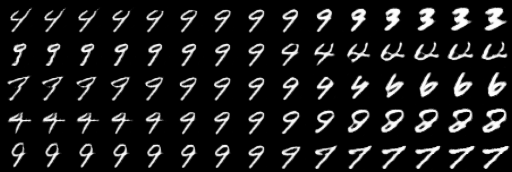GAN实现数据的从无到有是通过随机噪声z作为输入，在生成对抗下实现逼真数据的生成。这个过程是从全局的角度上直接生成数据样本， 很难分析具体的z对生成带来的影响。我们只知道在随机噪声z的作用下生成的数据样本和真实数据样本在分布上是随着网络的优化而逐渐相近的。为了进一步刻画数据的几何特性和数据样本的流形。 由齐国君团队在2018 CVPR上发表的Localized GAN通过局部坐标系来参数化不同位置的数据变换的局部几何， 缓解了GAN生成多样性不足的同时，还实现了在半监督分类的优化。

# 论文引入

• 通过局部坐标$z$可以实现数据的几何特性探索
• 论文在局部切线上施加正交性，缓解了生成多样性不足问题
• 给定一个图像x，用局部表示G(x,z)可以对这个x在它的局部领域中做各种编辑操作或者控制图像的各种属性
• 可以实现半监督分类任务的优化

# Localized GAN实现

Localized GAN创建的局部坐标系是实现算法的关键，如何去构造这个局部坐标系呢？我们通过图例分析：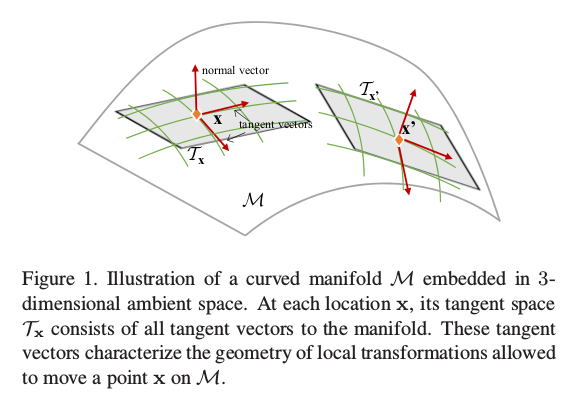$\begin{equation} \tau_x^j \triangleq \frac{\partial G(x,z)}{\partial z^j} \vert_{z=0} \in \mathbb R^D \end{equation}$

$\begin{equation} \langle \tau_x^i,\tau_x^j \rangle = \delta_{ij} \end{equation}$

(1)局部性：$G(x,0) = x$

(2)正交性：$J_x^T J_x = I_N$，其中$I_N$是大小为N的单位矩阵。

$\begin{equation} \Omega_{G(x)} = \mu \Vert G(x,0) -x \Vert^2 + \eta \Vert J_x^T J_x - I_N \Vert^2 \end{equation}$

# 训练Localized GAN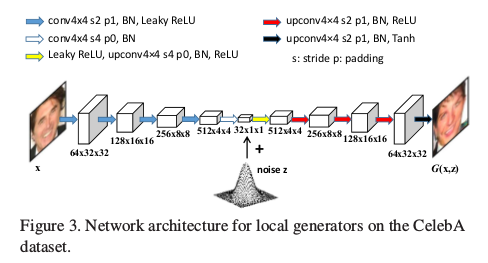$\begin{equation} \max_D \mathbb E_{x \sim P_x} log D(x) + \mathbb E_{x \sim P_x,z \sim P_z} log(1-D(G(x,z))) \end{equation}$

$\begin{equation} \min_G -\mathbb E_{x \sim P_x,z \sim P_z} log D(G(x,z)) + \mathbb E_{x \sim P_x} \Omega_G(x) \end{equation}$

# Localized GAN实验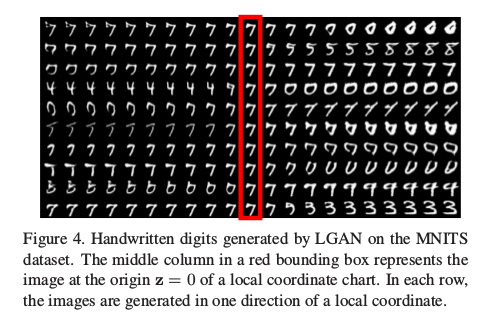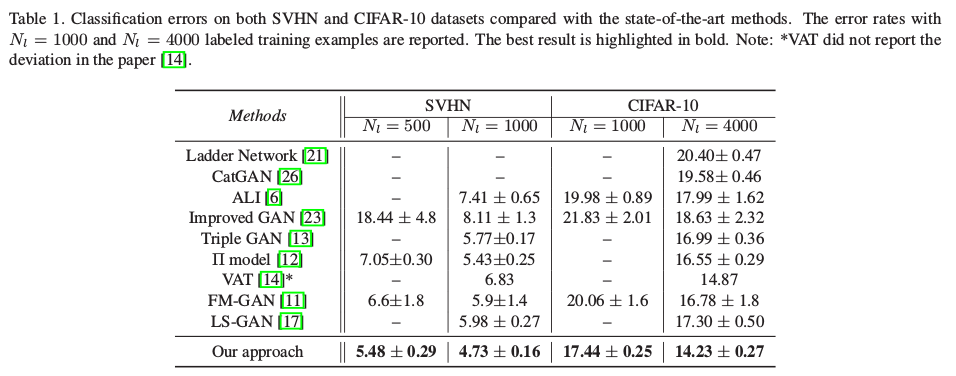LGAN_pytorch